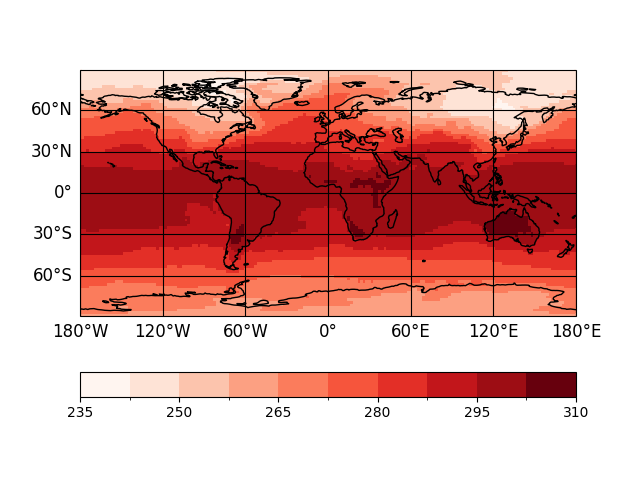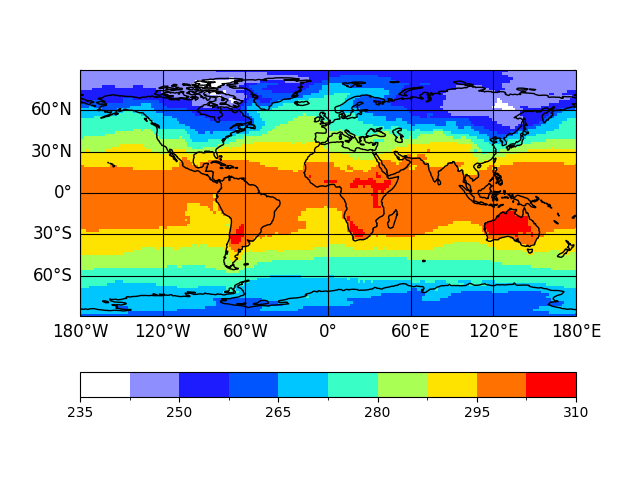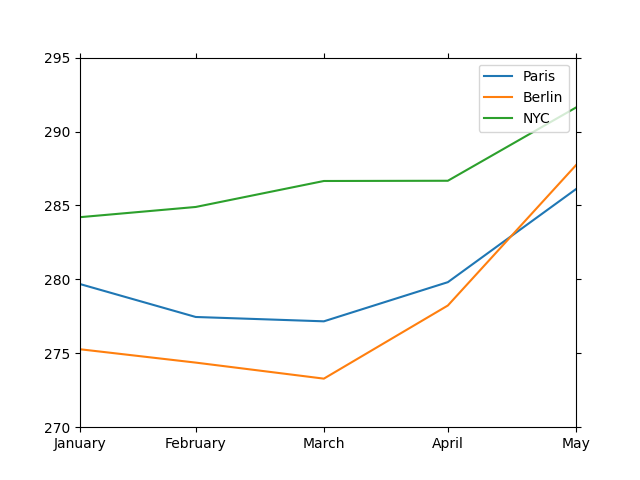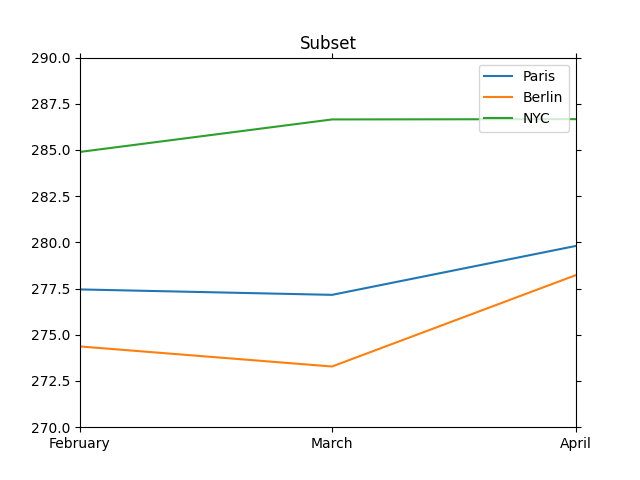# xarray Accessors

psyplot defines a `DataArray` and a `Dataset` accessor. You can use these accessors (see xarray Internals) to visualize your data and to update your plots. The following sections will show you how to make and update plots with these accessors. The plotmethods of the accessors are the same as for the `psyplot.project.plot` object.

## The `DatasetAccessor` dataset accessor

Importing the psyplot package registers a new dataset accessor (see `xarray.register_dataset_accessor()`), the `DatasetAccessor`. You can access it via the `psy` attribute of the `Dataset` class, i.e.

 `xarray.Dataset.psy`

It can be used to visualize the variables in the dataset directly from the dataset itself, e.g.

```In : import psyplot

In : ds = psyplot.open_dataset('demo.nc')

In : sp = ds.psy.plot.mapplot(name='t2m', cmap='Reds')
```The variable `sp` is a psyplot subproject of the current main project.

```In : print(sp)
psyplot.project.Project([    arr0: 2-dim DataArray of t2m, with (lat, lon)=(96, 192), lev=1e+05, time=1979-01-31T18:00:00])
```

Hence, it would be completely equivalent if you type

```In : import psyplot.project as psyplot

In : sp = psy.plot.mapplot(ds, name='t2m', cmap='Reds')
```

Note that the `DatasetAccessor.plot` attribute has the same plotmethods as the `psyplot.project.plot` instance.

## The `InteractiveArray` dataarray accessor

More advanced then the dataset accessor is the registered DataArray accessor, the `InteractiveArray`.

As well as the `DatasetAccessor`, it is registered as the `'psy'` attribute of any `DataArray`, i.e.

 `xarray.DataArray.psy`

You can use it for two things:

1. create plots of the array

2. update the plots and the array

### Creating plots with the dataarray accessor

Just use the `plot` attribute the accessor.

```In : import psyplot

In : ds = psyplot.open_dataset('demo.nc')

In : da = ds.t2m[0, 0]

# this is a two dimensional array
In : print(da)
<xarray.DataArray 't2m' (lat: 96, lon: 192)>
array([[251.41689, 251.454  , 251.48915, ..., 251.29774, 251.33876, 251.37978],
[254.16493, 254.33095, 254.50087, ..., 253.54774, 253.76845, 253.96376],
[255.86024, 256.3114 , 256.72742, ..., 254.40712, 254.90517, 255.42665],
...,
[263.70984, 263.6454 , 263.58875, ..., 263.96375, 263.86804, 263.78406],
[262.4989 , 262.48718, 262.47742, ..., 262.5536 , 262.5321 , 262.51453],
[260.8485 , 260.8661 , 260.88367, ..., 260.79578, 260.81335, 260.83093]],
dtype=float32)
Coordinates:
* lon      (lon) float64 0.0 1.875 3.75 5.625 7.5 ... 352.5 354.4 356.2 358.1
* lat      (lat) float64 88.57 86.72 84.86 83.0 ... -83.0 -84.86 -86.72 -88.57
lev      float64 1e+05
time     datetime64[ns] 1979-01-31T18:00:00
Attributes:
long_name:  Temperature
units:      K
code:       130
table:      128
grid_type:  gaussian

# and we can plot it using the mapplot plot method
In : plotter = da.psy.plot.mapplot()
```The resulting plotter, an instance of the `psyplot.plotter.Plotter` class, is the object that visualizes the data array. It can also be accessed via the `da.psy.plotter` attribute. Note that the creation of such a plotter overwrites any previous plotter in the `da.psy.plotter` attribute.

This methodology does not only work for `DataArrays`, but also for multiple DataArrays in a `InteractiveList`. This data structure is, for example, used by the `psyplot.project.plot.lineplot` plot method to visualize multiple lines. Consider the following example:

```In : ds0 = ds.isel(lev=0)  # select a subset of the dataset

# create a list of arrays at different longitudes
In : l = psyplot.InteractiveList([
....:     ds0.t2m.sel(lon=2.35, lat=48.86, method='nearest'),  # Paris
....:     ds0.t2m.sel(lon=13.39, lat=52.52, method='nearest'),  # Berlin
....:     ds0.t2m.sel(lon=-74.01, lat=40.71, method='nearest'),  # NYC
....:     ])
....:

In : l.arr_names = ['Paris', 'Berlin', 'NYC']

# plot the list
In : plotter = l.psy.plot.lineplot(xticks='data', xticklabels='%B')
```Note that for the `InteractiveList`, the `psy` attribute is just the list it self. So it would have been equivalent to call

```In : l.plot.lineplot()
```

### Updating plots and arrays with the dataarray accessor

The `InteractiveArray` accessor is designed for interactive usage of, not only the matplotlib figures, but also of the data. If you selected a subset of a dataset, e.g. via

```In : da = ds.t2m[0, 0]
....: print(da.time) # January 1979
....:
<xarray.DataArray 'time' ()>
array('1979-01-31T18:00:00.000000000', dtype='datetime64[ns]')
Coordinates:
lev      float64 1e+05
time     datetime64[ns] 1979-01-31T18:00:00
Attributes:
standard_name:  time
```

You can change to a different slice using the `InteractiveArray.update()` method.

```In : da.psy.base = ds   # tell psyplot the source of the dataarray

In : da.psy.update(time=2)
....: print(da.time)  # changed to March 1979
....:
<xarray.DataArray 'time' ()>
array('1979-03-31T18:00:00.000000000', dtype='datetime64[ns]')
Coordinates:
lev      float64 1e+05
time     datetime64[ns] 1979-03-31T18:00:00
Attributes:
standard_name:  time
```

The `da.psy.base = ds` command hereby tells the dataarray, where it is coming from, since this information is not known in the standard xarray framework.

Hint

You can avoid this, using the `DatasetAccessor.create_list()` method of the dataset accessor

```In : da = ds.psy.create_list(time=0, lev=0, name='t2m')
....: print(da.psy.base is ds)
....:
True
```

If you plotted the data, you can also change the formatoptions using the `update()` method, e.g.

```# create plot
In : da.psy.plot.mapplot();
``````In : da.psy.update(cmap='Reds')
```The same holds for the Interactive list

```In : l.update(time=slice(1, 4),  # change the data by selecting a subset of the timeslice
....:          title='Subset',    # change a formatoption, the title of the plot
....:          )
....:
```